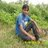16 Oct 2015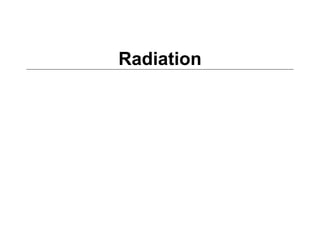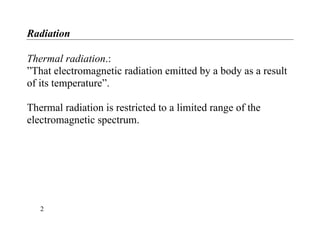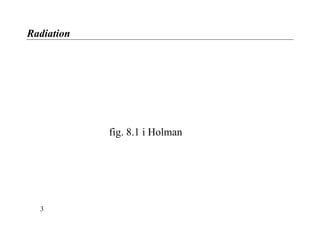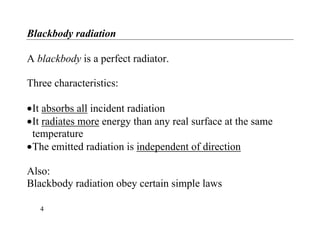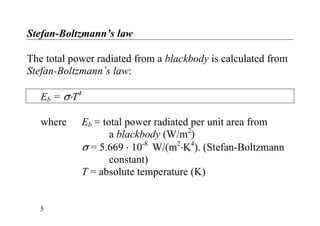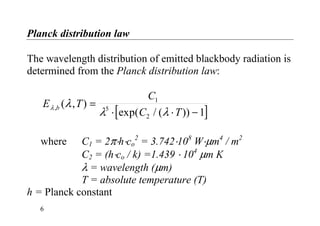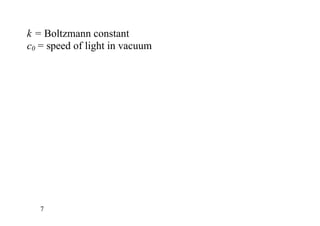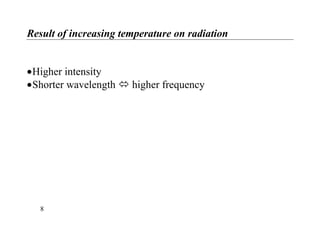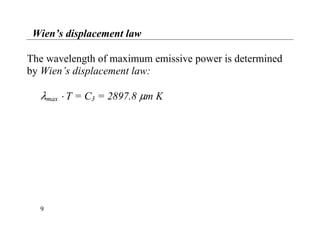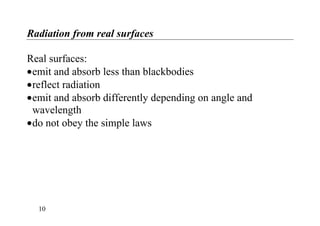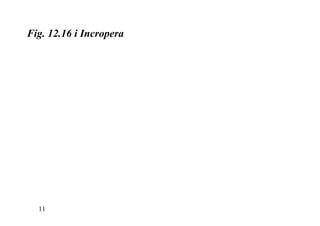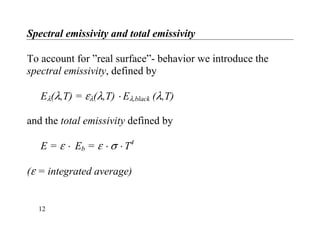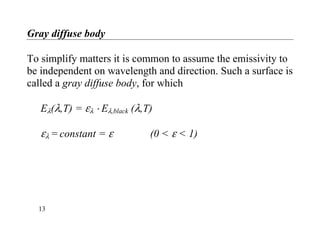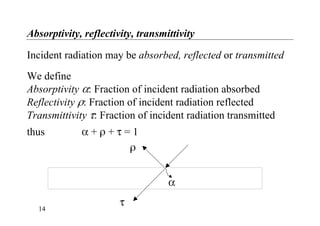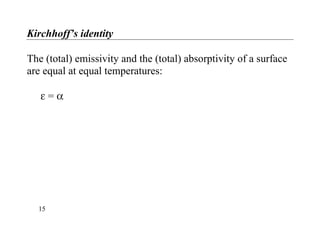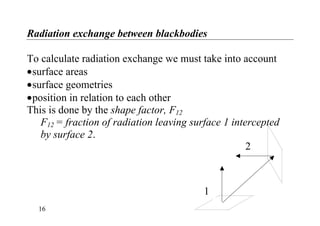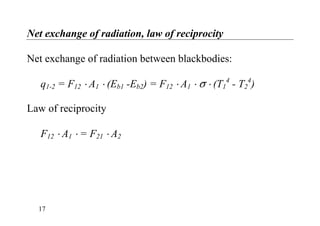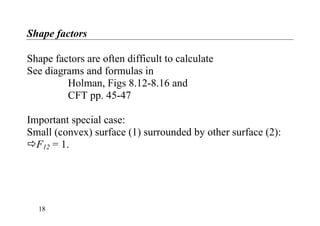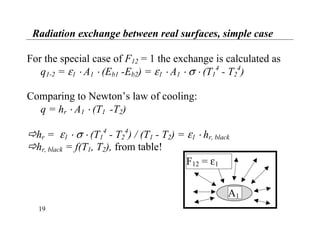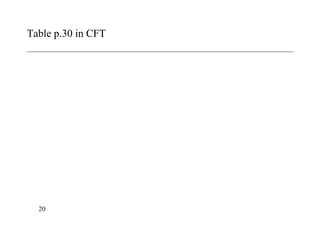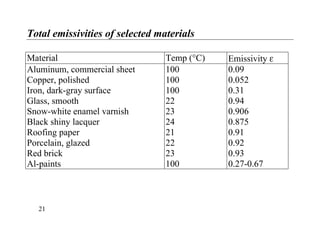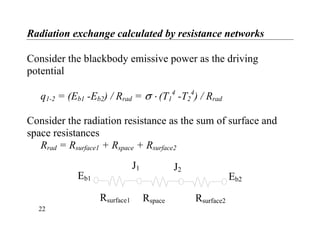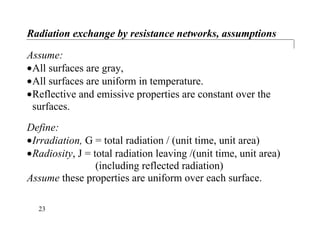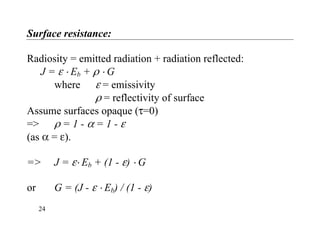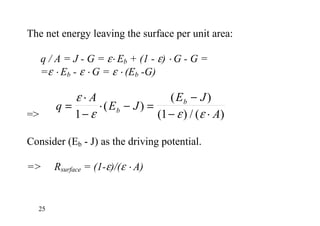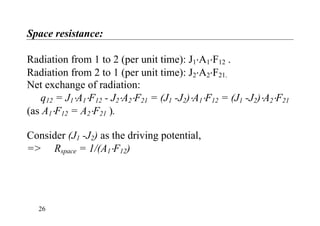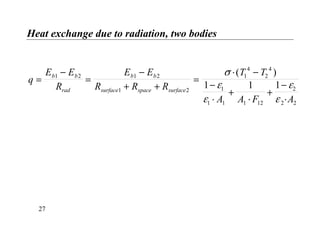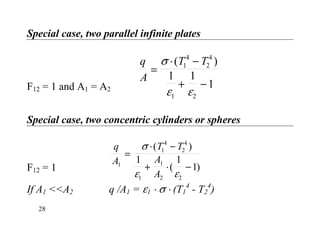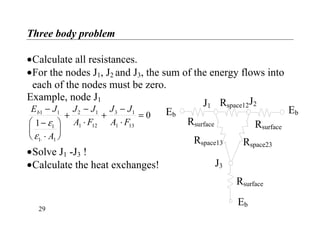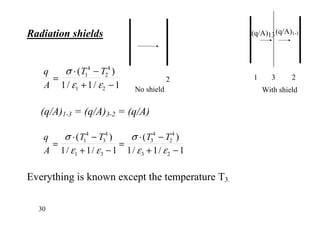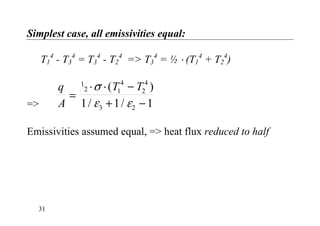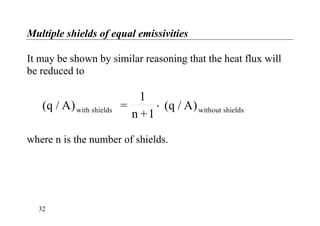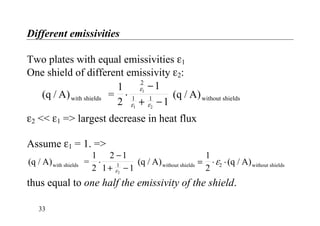1 sur 33

• 2. Radiation Thermal radiation.: ”That electromagnetic radiation emitted by a body as a result of its temperature”. Thermal radiation is restricted to a limited range of the electromagnetic spectrum. 2
• 4. Blackbody radiation A blackbody is a perfect radiator. Three characteristics: •It absorbs all incident radiation •It radiates more energy than any real surface at the same temperature •The emitted radiation is independent of direction Also: Blackbody radiation obey certain simple laws 4
• 5. Stefan-Boltzmann’s law The total power radiated from a blackbody is calculated from Stefan-Boltzmann´s law: Eb = σ⋅T4 where Eb = total power radiated per unit area from a blackbody (W/m2 ) σ = 5.669 ⋅ 10-8 W/(m2 ⋅K4 ). (Stefan-Boltzmann constant) T = absolute temperature (K) 5
• 6. Planck distribution law The wavelength distribution of emitted blackbody radiation is determined from the Planck distribution law: [ ] E T C C T bλ λ λ λ , ( , ) exp( / ( )) = ⋅ ⋅ 1 5 2 1− where C1 = 2π⋅h⋅co 2 = 3.742⋅108 W⋅µm4 / m2 C2 = (h⋅co / k) =1.439 ⋅ 104 µm K λ = wavelength (µm) T = absolute temperature (T) h = Planck constant 6
• 7. k = Boltzmann constant c0 = speed of light in vacuum 7
• 8. Result of increasing temperature on radiation •Higher intensity •Shorter wavelength higher frequency 8
• 9. Wien’s displacement law The wavelength of maximum emissive power is determined by Wien’s displacement law: λmax ⋅ T = C3 = 2897.8 µm K 9
• 10. Radiation from real surfaces Real surfaces: •emit and absorb less than blackbodies •reflect radiation •emit and absorb differently depending on angle and wavelength •do not obey the simple laws 10
• 11. Fig. 12.16 i Incropera 11
• 12. Spectral emissivity and total emissivity To account for ”real surface”- behavior we introduce the spectral emissivity, defined by Eλ(λ,T) = ελ(λ,T) ⋅ Eλ,black (λ,T) and the total emissivity defined by E = ε ⋅ Eb = ε ⋅ σ ⋅ T4 (ε = integrated average) 12
• 13. Gray diffuse body To simplify matters it is common to assume the emissivity to be independent on wavelength and direction. Such a surface is called a gray diffuse body, for which Eλ(λ,T) = ελ ⋅ Eλ,black (λ,T) ελ = constant = ε (0 < ε < 1) 13
• 14. Absorptivity, reflectivity, transmittivity Incident radiation may be absorbed, reflected or transmitted We define Absorptivity α: Fraction of incident radiation absorbed Reflectivity ρ: Fraction of incident radiation reflected Transmittivity τ: Fraction of incident radiation transmitted thus α + ρ + τ = 1 14 τ α ρ
• 15. Kirchhoff’s identity The (total) emissivity and the (total) absorptivity of a surface are equal at equal temperatures: ε = α 15
• 16. Radiation exchange between blackbodies To calculate radiation exchange we must take into account •surface areas •surface geometries •position in relation to each other This is done by the shape factor, F12 F12 = fraction of radiation leaving surface 1 intercepted 1 2 by surface 2. 16
• 17. Net exchange of radiation, law of reciprocity Net exchange of radiation between blackbodies: q1-2 = F12 ⋅ A1 ⋅ (Eb1 -Eb2) = F12 ⋅ A1 ⋅ σ ⋅ (T1 4 - T2 4 ) Law of reciprocity F12 ⋅ A1 ⋅ = F21 ⋅ A2 17
• 18. Shape factors Shape factors are often difficult to calculate See diagrams and formulas in Holman, Figs 8.12-8.16 and CFT pp. 45-47 Important special case: Small (convex) surface (1) surrounded by other surface (2): F12 = 1. 18
• 19. Radiation exchange between real surfaces, simple case For the special case of F12 = 1 the exchange is calculated as q1-2 = ε1 ⋅ A1 ⋅ (Eb1 -Eb2) = ε1 ⋅ A1 ⋅ σ ⋅ (T1 4 - T2 4 ) Comparing to Newton’s law of cooling: q = hr ⋅ A1 ⋅ (T1 -T2) hr = ε1 ⋅ σ ⋅ (T1 4 - T2 4 ) / (T1 - T2) = ε1 ⋅ hr, black hr, black = f(T1, T2), from table! A1 F12 = ε1 19
• 20. Table p.30 in CFT 20
• 21. Total emissivities of selected materials Material Temp (°C) Emissivity ε Aluminum, commercial sheet 100 0.09 Copper, polished 100 0.052 Iron, dark-gray surface 100 0.31 Glass, smooth 22 0.94 Snow-white enamel varnish 23 0.906 Black shiny lacquer 24 0.875 Roofing paper 21 0.91 Porcelain, glazed 22 0.92 Red brick 23 0.93 Al-paints 100 0.27-0.67 21
• 22. Radiation exchange calculated by resistance networks Consider the blackbody emissive power as the driving potential q1-2 = (Eb1 -Eb2) / Rrad = σ ⋅ (T1 4 -T2 4 ) / Rrad Consider the radiation resistance as the sum of surface and space resistances Rrad = Rsurface1 + Rspace + Rsurface2 J2J1 Eb1 Eb2 Rsurface1 Rspace Rsurface2 22
• 23. Radiation exchange by resistance networks, assumptions Assume: •All surfaces are gray, •All surfaces are uniform in temperature. •Reflective and emissive properties are constant over the surfaces. Define: •Irradiation, G = total radiation / (unit time, unit area) •Radiosity, J = total radiation leaving /(unit time, unit area) (including reflected radiation) Assume these properties are uniform over each surface. 23
• 24. Surface resistance: Radiosity = emitted radiation + radiation reflected: J = ε ⋅ Eb + ρ ⋅ G where ε = emissivity ρ = reflectivity of surface Assume surfaces opaque (τ=0) => ρ = 1 - α = 1 - ε (as α = ε). => J = ε⋅ Eb + (1 - ε) ⋅ G or G = (J - ε ⋅ Eb) / (1 - ε) 24
• 25. The net energy leaving the surface per unit area: q / A = J - G = ε⋅ Eb + (1 - ε) ⋅ G - G = =ε ⋅ Eb - ε ⋅ G = ε ⋅ (Eb -G) => q A E J E J Ab b = ⋅ − ⋅ − = − − ⋅ ε ε ε ε1 1 ( ) ( ) ( ) / ( ) Consider (Eb - J) as the driving potential. => Rsurface = (1-ε)/(ε ⋅ A) 25
• 26. Space resistance: Radiation from 1 to 2 (per unit time): J1⋅A1⋅F12 . Radiation from 2 to 1 (per unit time): J2⋅A2⋅F21. Net exchange of radiation: q12 = J1⋅A1⋅F12 - J2⋅A2⋅F21 = (J1 -J2)⋅A1⋅F12 = (J1 -J2)⋅A2⋅F21 (as A1⋅F12 = A2⋅F21 ). Consider (J1 -J2) as the driving potential, => Rspace = 1/(A1⋅F12) 26
• 27. Heat exchange due to radiation, two bodies q E E R E E R R R T T A A F A b b rad b b surface space surface = − = − + + = ⋅ − − ⋅ + ⋅ + − ⋅ 1 2 1 2 1 2 1 4 2 4 1 1 1 1 12 2 2 2 1 1 1 σ ε ε ε ε ( ) 27
• 28. Special case, two parallel infinite plates F12 = 1 and A1 = A2 q A T T = ⋅ − + − σ ε ε ( )1 4 2 4 1 2 1 1 1 Special case, two concentric cylinders or spheres F12 = 1 q A T T A A 1 1 4 2 4 1 1 2 2 1 1 1 = ⋅ − + ⋅ − σ ε ε ( ) ( ) If A1 <<A2 q /A1 = ε1 ⋅ σ ⋅ (T1 4 - T2 4 ) 28
• 29. Three body problem •Calculate all resistances. •For the nodes J1, J2 and J3, the sum of the energy flows into each of the nodes must be zero. Example, node J1 E J J J− − J2J1 J3 Eb Rspace12 Rspace23Rspace13 Rsurface Rsurface Rsurface A A F J J A F b1 1 1 1 1 2 1 1 12 3 1 1 131 0 − ⋅       + ⋅ + − ⋅ = ⋅ ε ε EbEb •Solve J1 -J3 ! •Calculate the heat exchanges! 29
• 30. Radiation shields q A T T = ⋅ − + − σ ε ε ( ) / / 1 4 2 4 1 21 1 1 1 2 No shield 1 23 With shield (q/A)1-3(q/A)13 (q/A)1-3 = (q/A)3-2 = (q/A) q A T T T T = ⋅ − + − = ⋅ − + − σ ε ε σ ε ε ( ) / / ( ) / / 1 4 3 4 1 3 3 4 2 4 3 21 1 1 1 1 1 Everything is known except the temperature T3. 30
• 31. Simplest case, all emissivities equal: T1 4 - T3 4 = T3 4 - T2 4 => T3 4 = ½ ⋅ (T1 4 + T2 4 ) => q A T T = ⋅ ⋅ − + − 1 2 1 4 2 4 3 21 1 1 σ ε ε ( ) / / Emissivities assumed equal, => heat flux reduced to half 31
• 32. Multiple shields of equal emissivities It may be shown by similar reasoning that the heat flux will be reduced to (q / A) = 1 n +1 (q / A)with shields without shields⋅ where n is the number of shields. 32
• 33. 33 Different emissivities Two plates with equal emissivities ε1 One shield of different emissivity ε2: 1 2 −1 (q / A) = 2 (q / A)with shields without shields 1 ⋅ + − ε ε ε 11 1 1 2 ε2 << ε1 => largest decrease in heat flux Assume ε1 = 1. => 1 (q / A) = 2 (q / A) (q / A)with shields without shields without shields⋅ − + − = ⋅ ⋅ 2 1 1 1 1 21 2 2ε ε thus equal to one half the emissivity of the shield.